Скачать презентацию A linear time algorithm for the weighted lexicographic

4ca1055454de277218435fea9bbde916.ppt

• Количество слайдов: 19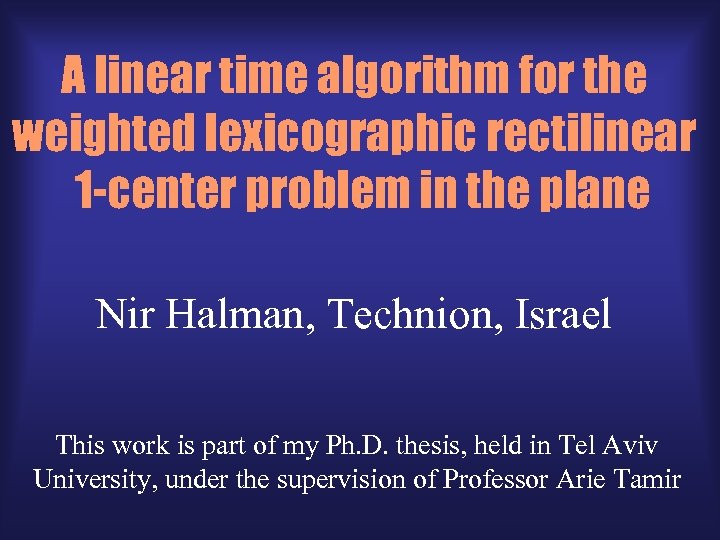A linear time algorithm for the weighted lexicographic rectilinear 1 -center problem in the plane Nir Halman, Technion, Israel This work is part of my Ph. D. thesis, held in Tel Aviv University, under the supervision of Professor Arie Tamir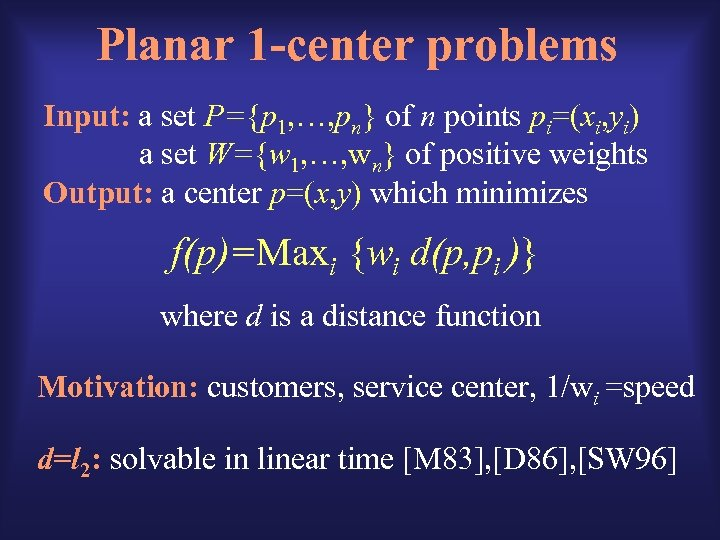Planar 1 -center problems Input: a set P={p 1, …, pn} of n points pi=(xi, yi) a set W={w 1, …, wn} of positive weights Output: a center p=(x, y) which minimizes f(p)=Maxi {wi d(p, pi )} where d is a distance function Motivation: customers, service center, 1/wi =speed d=l 2: solvable in linear time [M 83], [D 86], [SW 96]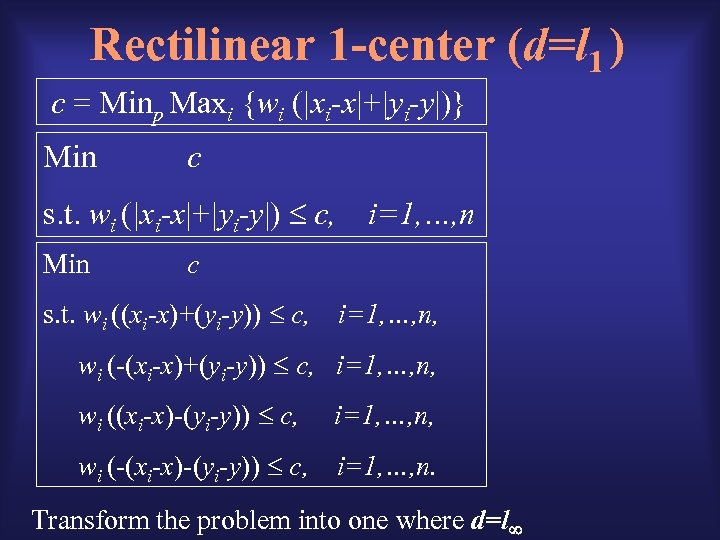Rectilinear 1 -center (d=l 1 ) c = Minp Maxi {wi (|xi-x|+|yi-y|)} Min c s. t. wi (|xi-x|+|yi-y|) c, Min i=1, …, n c s. t. wi ((xi-x)+(yi-y)) c, i=1, …, n, wi (-(xi-x)+(yi-y)) c, i=1, …, n, wi ((xi-x)-(yi-y)) c, i=1, …, n, wi (-(xi-x)-(yi-y)) c, i=1, …, n. Transform the problem into one where d=l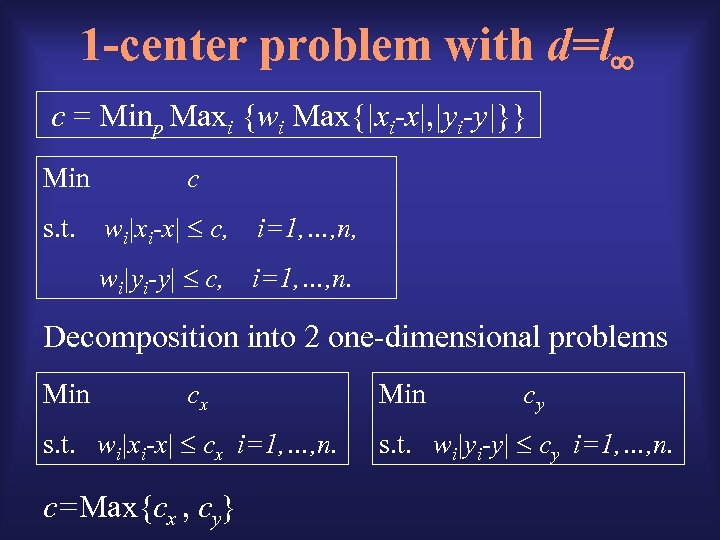1 -center problem with d=l c = Minp Maxi {wi Max{|xi-x|, |yi-y|}} Min c wi|xi-x| c, i=1, …, n, wi|yi-y| c, s. t. i=1, …, n. Decomposition into 2 one-dimensional problems Min cx s. t. wi|xi-x| cx i=1, …, n. c=Max{cx , cy} Min cy s. t. wi|yi-y| cy i=1, …, n.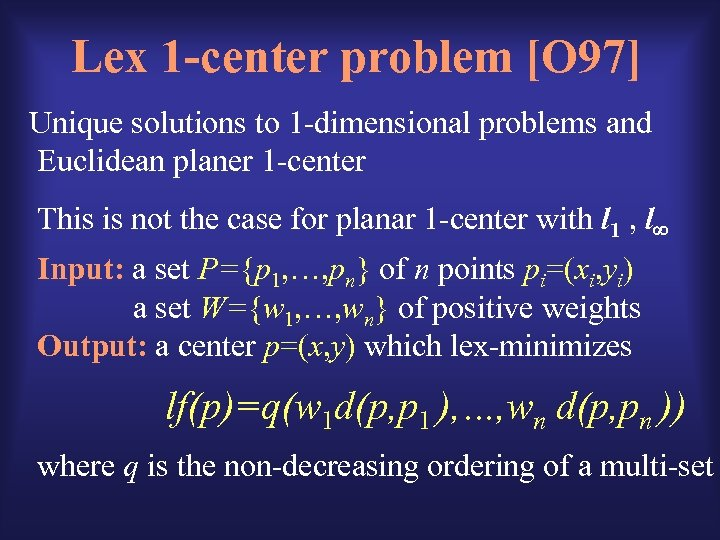Lex 1 -center problem [O 97] Unique solutions to 1 -dimensional problems and Euclidean planer 1 -center This is not the case for planar 1 -center with l 1 , l Input: a set P={p 1, …, pn} of n points pi=(xi, yi) a set W={w 1, …, wn} of positive weights Output: a center p=(x, y) which lex-minimizes lf(p)=q(w 1 d(p, p 1 ), …, wn d(p, pn )) where q is the non-decreasing ordering of a multi-set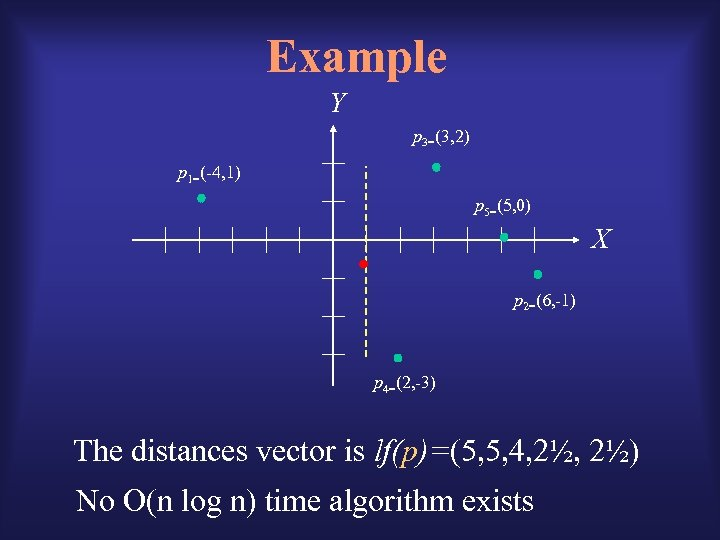Example Y p 3=(3, 2) p 1=(-4, 1) p 5=(5, 0) X p 2=(6, -1) p 4=(2, -3) The distances vector is lf(p)=(5, 5, 4, 2½) No O(n log n) time algorithm exists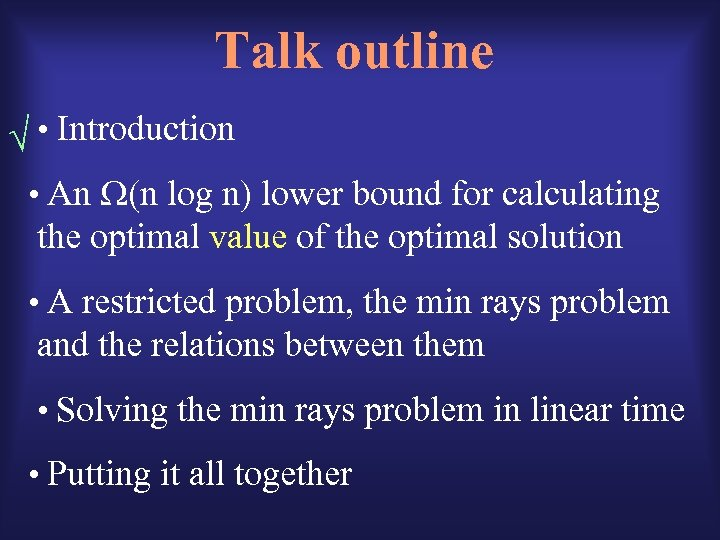Talk outline • Introduction • An (n log n) lower bound for calculating the optimal value of the optimal solution • A restricted problem, the min rays problem and the relations between them • Solving the min rays problem in linear time • Putting it all together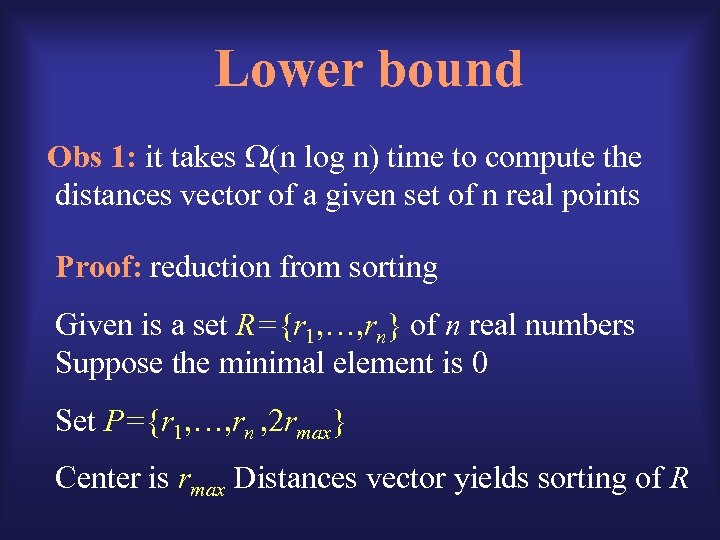Lower bound Obs 1: it takes (n log n) time to compute the distances vector of a given set of n real points Proof: reduction from sorting Given is a set R={r 1, …, rn} of n real numbers Suppose the minimal element is 0 Set P={r 1, …, rn , 2 rmax} Center is rmax Distances vector yields sorting of R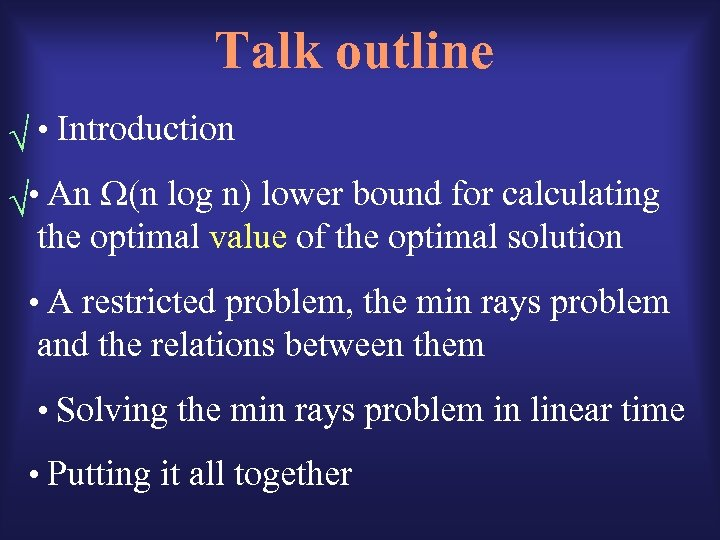Talk outline • Introduction • An (n log n) lower bound for calculating the optimal value of the optimal solution • A restricted problem, the min rays problem and the relations between them • Solving the min rays problem in linear time • Putting it all together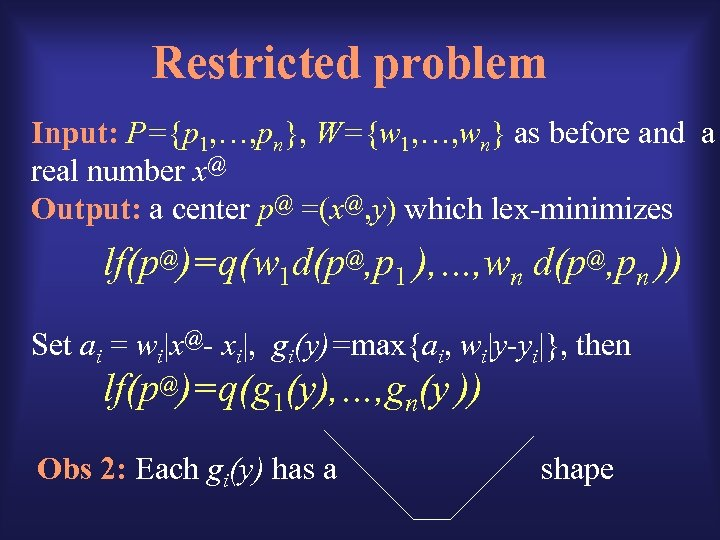Restricted problem Input: P={p 1, …, pn}, W={w 1, …, wn} as before and a real number [email protected] Output: a center [email protected] =([email protected], y) which lex-minimizes lf([email protected])=q(w 1 d([email protected], p 1 ), …, wn d([email protected], pn )) Set ai = wi|[email protected] xi|, gi(y)=max{ai, wi|y-yi|}, then lf([email protected])=q(g 1(y), …, gn(y )) Obs 2: Each gi(y) has a shape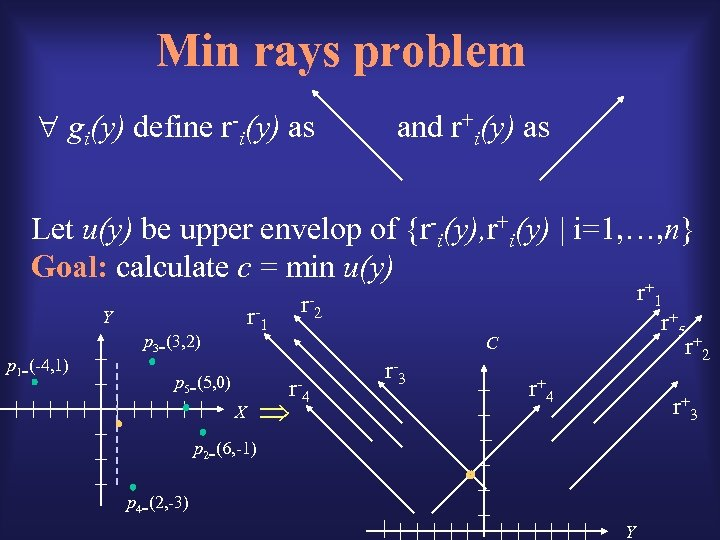Min rays problem gi(y) define r-i(y) as and r+i(y) as Let u(y) be upper envelop of {r-i(y), r+i(y) | i=1, …, n} Goal: calculate c = min u(y) + r- Y p 3=(3, 2) p 1=(-4, 1) p 5=(5, 0) X r r -2 1 r+ 5 r+ 2 C r -4 r -3 1 r+ 4 r+ 3 p 2=(6, -1) p 4=(2, -3) Y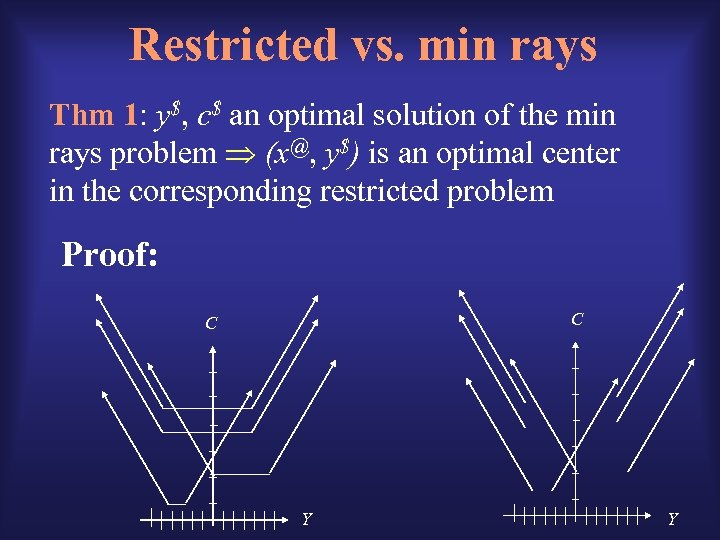Restricted vs. min rays Thm 1: y\$, c\$ an optimal solution of the min rays problem ([email protected], y\$) is an optimal center in the corresponding restricted problem Proof: C C Y Y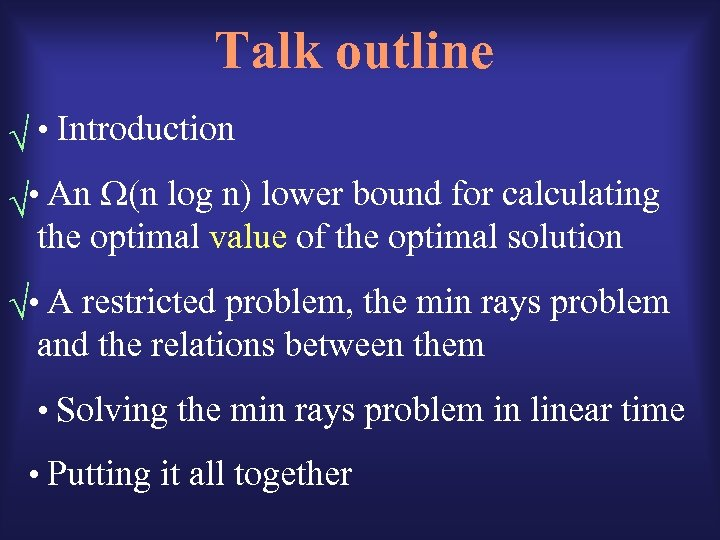Talk outline • Introduction • An (n log n) lower bound for calculating the optimal value of the optimal solution • A restricted problem, the min rays problem and the relations between them • Solving the min rays problem in linear time • Putting it all together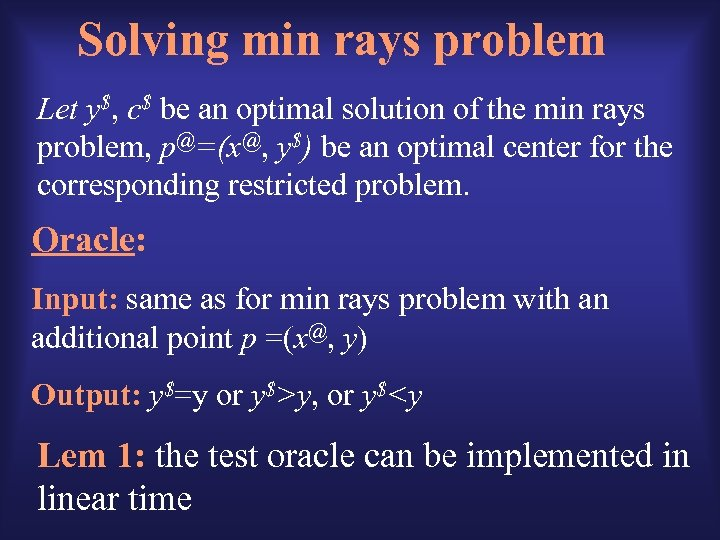Solving min rays problem Let y\$, c\$ be an optimal solution of the min rays problem, [email protected]=([email protected], y\$) be an optimal center for the corresponding restricted problem. Oracle: Input: same as for min rays problem with an additional point p =([email protected], y) Output: y\$=y or y\$>y, or y\$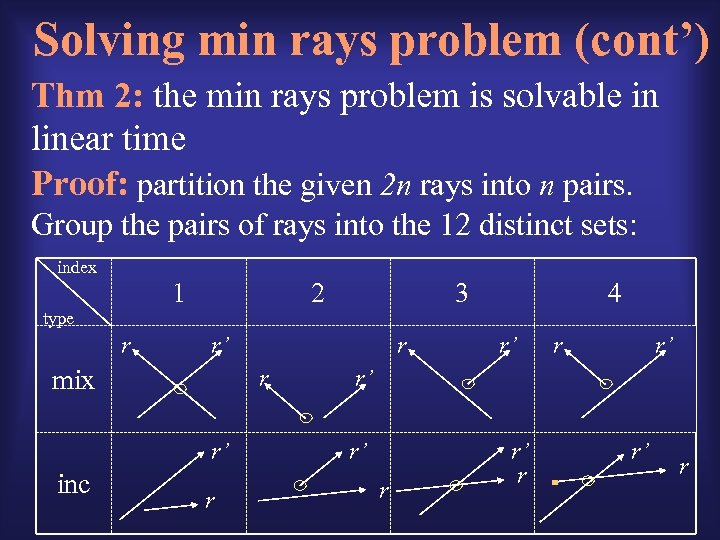Solving min rays problem (cont’) Thm 2: the min rays problem is solvable in linear time Proof: partition the given 2 n rays into n pairs. Group the pairs of rays into the 12 distinct sets: index 1 2 3 4 type r r’ mix r r’ inc r r r’ r’ r’ r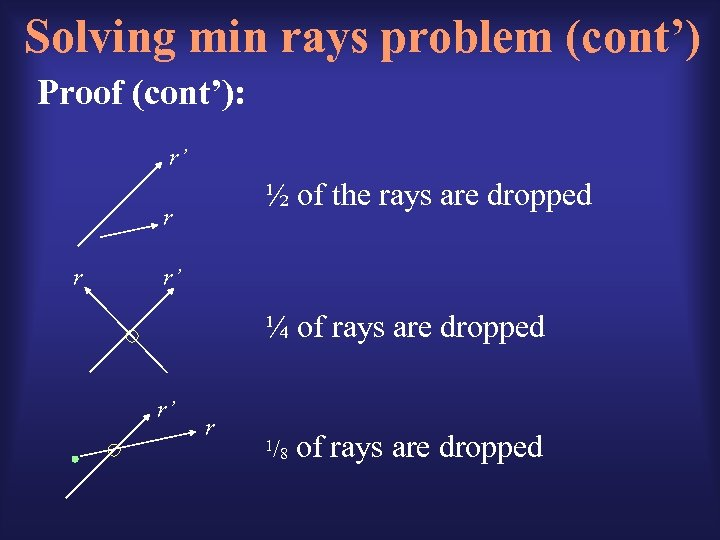Solving min rays problem (cont’) Proof (cont’): r’ ½ of the rays are dropped r r r’ ¼ of rays are dropped r’ r 1/ 8 of rays are dropped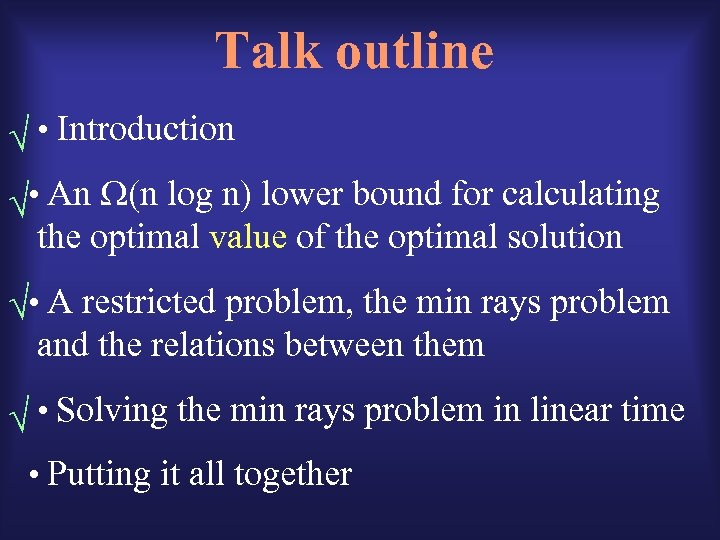Talk outline • Introduction • An (n log n) lower bound for calculating the optimal value of the optimal solution • A restricted problem, the min rays problem and the relations between them • Solving the min rays problem in linear time • Putting it all together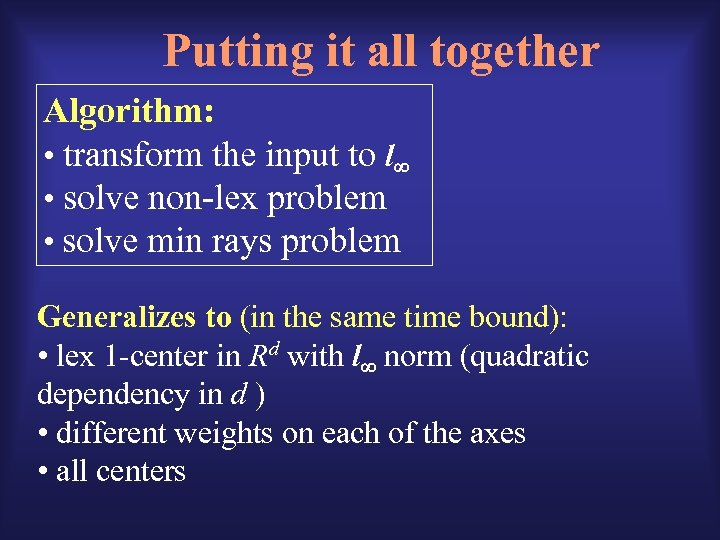Putting it all together Algorithm: • transform the input to l • solve non-lex problem • solve min rays problem Generalizes to (in the same time bound): • lex 1 -center in Rd with l norm (quadratic dependency in d ) • different weights on each of the axes • all centersThank you !